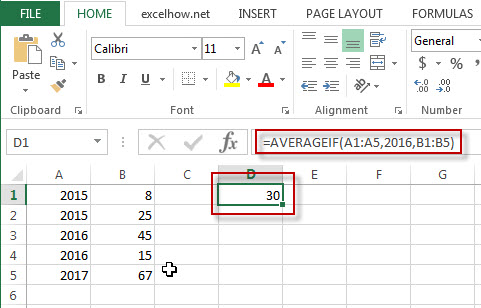# Excel AverageIF Function

This post will guide you how to use Excel AVERAGEIF function with syntax and examples in Microsoft excel.

### Description

The Excel AVERAGEAIF function returns the average of all numbers in a range of cells that meet a given criteria.

The AVERAGEIF function is a build-in function in Microsoft Excel and it is categorized as a Statistical Function.

The AVERAGEIF function is available in Excel 2016, Excel 2013, Excel 2010, Excel 2007, Excel 2003, Excel XP, Excel 2000, Excel 2011 for Mac.

### Syntax

The syntax of the AVERAGEIF function is as below:

`= AVERAGEIF (range, criteria, [average_range])`

Where the AVERAGEIF function arguments are:

• Range -This is a required argument.  The range of cells that you want to apply the criteria to average
• Criteria – This is a required argument.  The criteria used to define which cells are averaged
• Average_range – This is an optional argument.  The average range is actually to average. If it is omitted, the function will use Range values to average.

### Example

The below examples will show you how to use Excel AVERAGEIF Function to return the average of all numbers in a range of cells based on a given criteria.

#1 To get the average of all numbers in average range B1:B5 and meet the criteria that equal to “2016” in range A1:A5, and the, just using the following excel formula: =AVERAGEIF(A1:A5,2015,B1:B5)Related Posts

Excel VARP Function

This post will guide you how to use the VARP function with syntax and examples in Microsoft excel. Description The Excel VARP function returns the variance of a population based on an entire population of numbers. This function has been replaced ...

Excel VARA Function

This post will guide you how to use the VARA function with syntax and examples in Microsoft excel. Description The Excel VARA function returns the variance of a population based on a sample of numbers, text, or logical values. The variance ...

Excel VAR Function

This post will guide you how to use the VAR function with syntax and examples in Microsoft excel. Description The Excel VAR function returns the variance of a population based on a sample of numbers. This function has been replaced by ...

Excel STDEVPA Function

This post will guide you how to use the STDEVPA function with syntax and examples in Microsoft excel. Description The Excel STDEVPA function returns the standard deviation of a population based on an entire population of numbers, text or logical values. ...

Excel STDEVP Function

This post will guide you how to use the STDEVP function with syntax and examples in Microsoft excel. Description The Excel STDEVP function returns the standard deviation of a population based on an entire population of numbers. This function has been ...

Sidebar